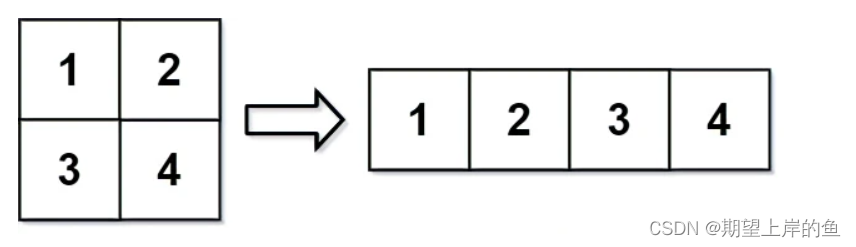## ( 数组和矩阵) 566. 重塑矩阵 ——【Leetcode每日一题】

2023/11/30 8:47:55

## ❓566. 重塑矩阵

#### 示例 1：#### 示例 2：• m == mat.length
• n == mat[i].length
• 1 <= m, n <= 100
• -1000 <= mat[i][j] <= 1000
• 1 <= r, c <= 300

### 💡思路：

{ r =  index  / n c =  index  % n \left\{\begin{array}{l}\mathrm{r}=\text { index } / \mathrm{n} \\\mathrm{c}=\text { index } \% \mathrm{n}\end{array}\right.

🍁代码：(Java、C++)
Java

class Solution {
public int[][] matrixReshape(int[][] mat, int r, int c) {
int m = mat.length, n = mat.length;
if(m * n != r * c) return mat;
int[][] ans = new int[r][c];
int index = 0;
for(int i = 0; i < r; i++){
for(int j = 0; j < c; j++){
ans[i][j] = mat[index / n][index % n];
index++;
}
}
return ans;
}
}


C++

class Solution {
public:
vector<vector<int>> matrixReshape(vector<vector<int>>& mat, int r, int c) {
int m = mat.size(), n = mat.size();
if(m * n != r * c) return mat;
vector<vector<int>> ans(r, vector<int>(c));
int index = 0;
for(int i = 0; i < r; i++){
for(int j = 0; j < c; j++){
ans[i][j] = mat[index / n][index % n];
index++;
}
}
return ans;
}
};


#### 🚀 运行结果：#### 🕔 复杂度分析：

• 时间复杂度 O ( r c ) O(rc) ，里的时间复杂度是在重塑矩阵成功的前提下的时间复杂度。
• 空间复杂度 O ( 1 ) O(1) ，这里的空间复杂度不包含返回的重塑矩阵需要的空间。

### Golang-常见数据结构Slice

Slice slice 翻译成中文就是切片&#xff0c;它和数组&#xff08;array&#xff09;很类似&#xff0c;可以用下标的方式进行访问&#xff0c;如果越界&#xff0c;就会产生 panic。但是它比数组更灵活&#xff0c;可以自动地进行扩容。 了解 slice 的本质, 最简单的方法就是…

### iOS开发之Masonry框架源码深度解析

Masonry是iOS在控件布局中经常使用的一个轻量级框架&#xff0c;Masonry让NSLayoutConstraint使用起来更为简洁。Masonry简化了NSLayoutConstraint的使用方式&#xff0c;让我们可以以链式的方式为我们的控件指定约束。本篇博客的主题不是教你如何去使用Masonry框架的&#xff…

### Small Tip: 怎么去Schedule一个Analysis for Office的workbook

workbook的query不能是本地的&#xff0c;也就是说不能是在包\$Tmp里面的。这种的没办法在BO里面用SSO。也就没办法Schedule。 前提条件有&#xff1a; 1.BO和BW系统的SSO配置得OK。 如果没有SSO&#xff0c;那么每次打开workbook就会有一个要登录BW的弹窗&#xff0c;这样是…